## 【C++】初步认识模板

article2023/9/25 14:12:18/

🏖️作者：@malloc不出对象
⛺专栏：C++的学习之路
👦个人简介：一名双非本科院校大二在读的科班编程菜鸟，努力编程只为赶上各位大佬的步伐🙈🙈### 目录

• 前言
• 一、泛型编程
• 二、函数模板
• 2.1 函数模板的原理
• 2.2 函数模板的实例化
• 2.3 模板参数的匹配原则
• 三、类模板
• 3.1 类模板的实例化
• 3.2 类模板与模板类

## 前言

### 一、泛型编程

``````void Swap(int& left, int& right)
{
int temp = left;
left = right;
right = temp;
}

void Swap(double& left, double& right)
{
double temp = left;
left = right;
right = temp;
}

void Swap(char& left, char& right)
{
char temp = left;
left = right;
right = temp;
}
// ......
``````

1.重载的函数仅仅是类型不同，代码复用率比较低，只要有新类型出现时，就需要用户自己增加对应的函数
2.代码的可维护性比较低，一个出错可能所有的重载均出错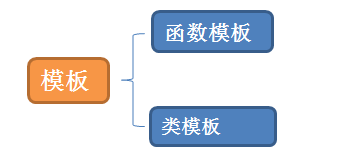### 二、函数模板

`template<typename T1, typename T2,......,typename Tn>`，同样也可以使用`template<class T1, class T2, ......, class Tn>`，关于这两种方式的区别我们后续再来谈。

``````#include <iostream>
using namespace std;

//template<class T>
template<typename T>
void Swap(T& a, T& b)
{
T tmp = a;
a = b;
b = tmp;
}

int main()
{
int a = 1, b = 2;
Swap(a, b);
cout << a << " " << b << endl;

double c = 1.2, d = 3.4;
Swap(c, d);
cout << c << " " << d << endl;

return 0;
}
``````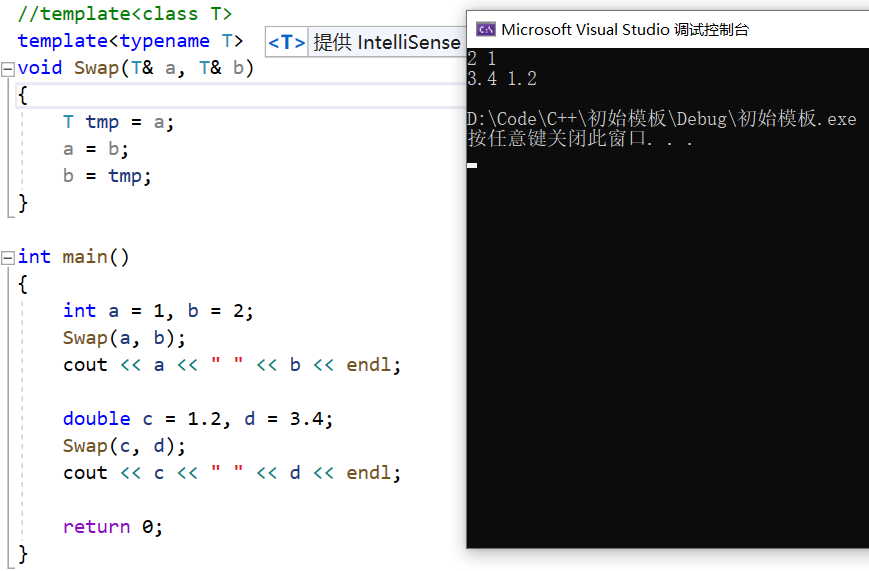#### 2.1 函数模板的原理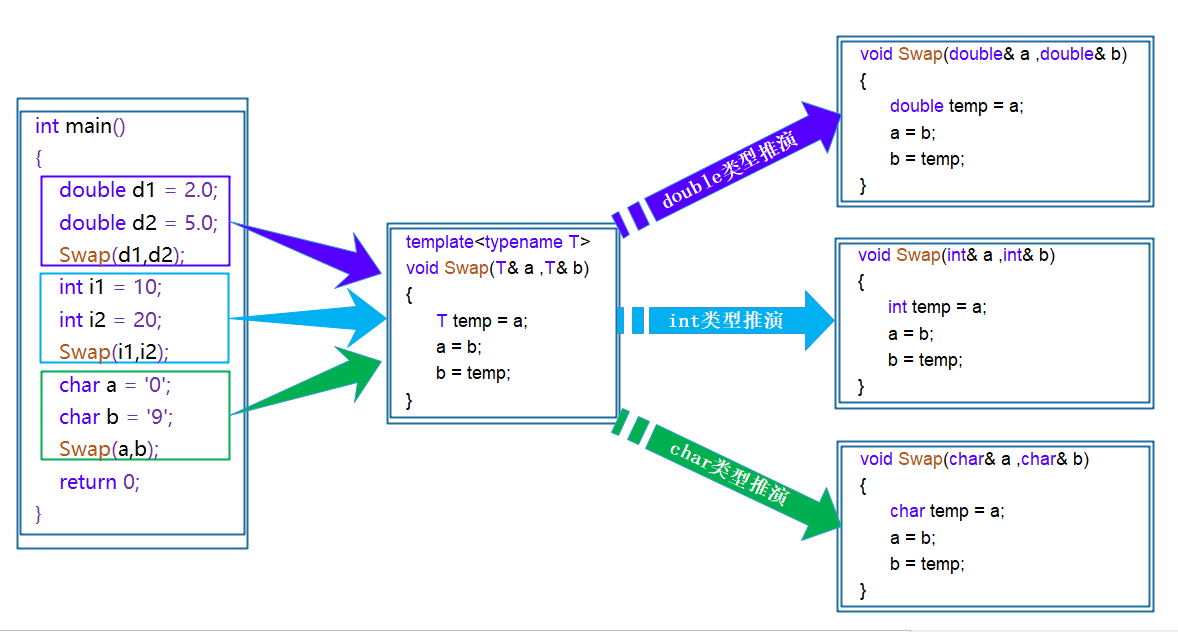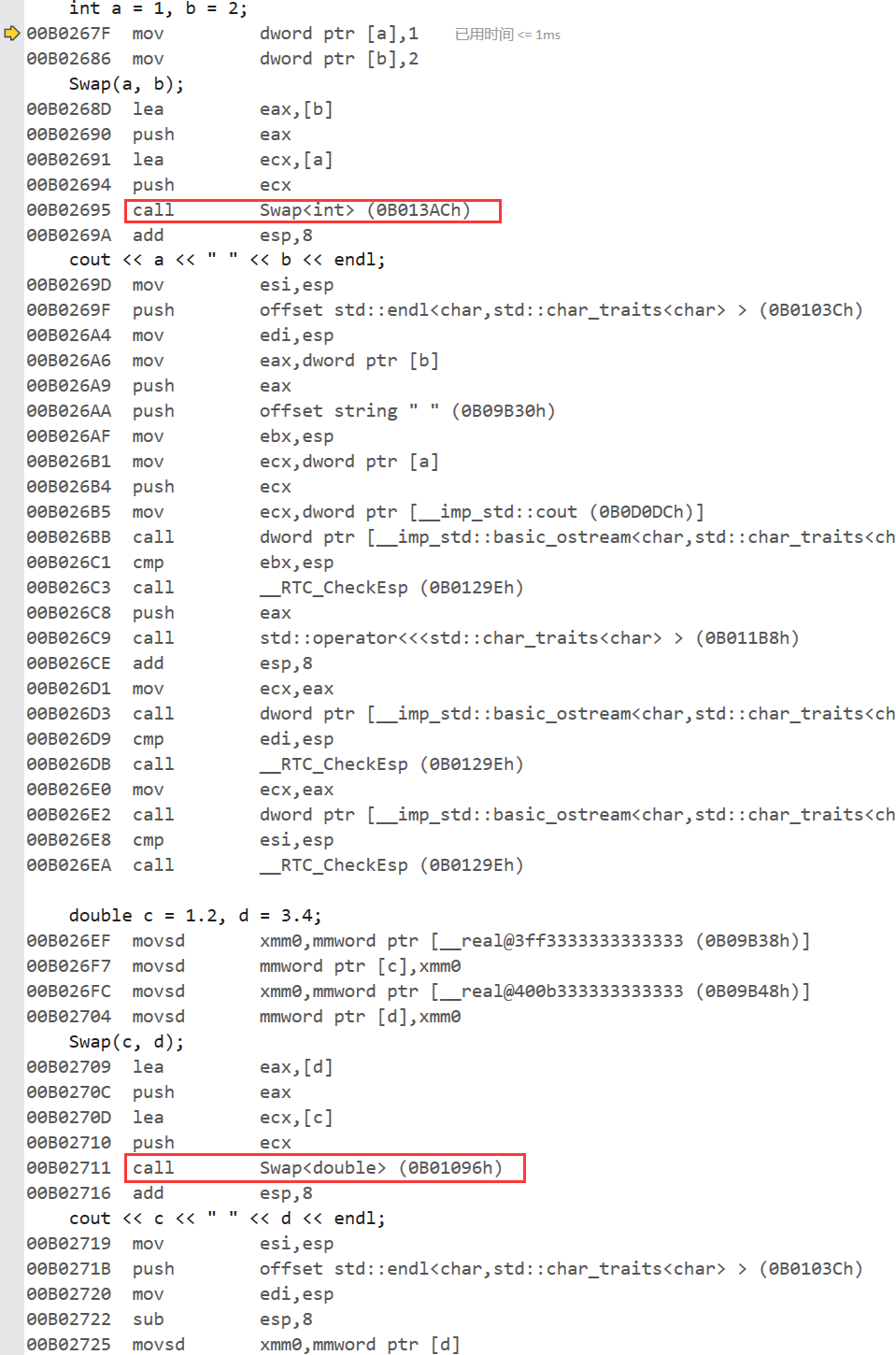#### 2.2 函数模板的实例化

• 隐式实例化：让编译器根据实参推演模板参数的实际类型
``````#include <iostream>
using namespace std;

template<class T>
T Add(const T& left, const T& right)
{
return left + right;
}

int main()
{
int a1 = 10, a2 = 20;
double d1 = 10.2, d2 = 20.3;

// 实参传递给形参，自动推演模板类型
cout << Add(a1, a2) << endl;
cout << Add(d1, d2) << endl;

return 0;
}
``````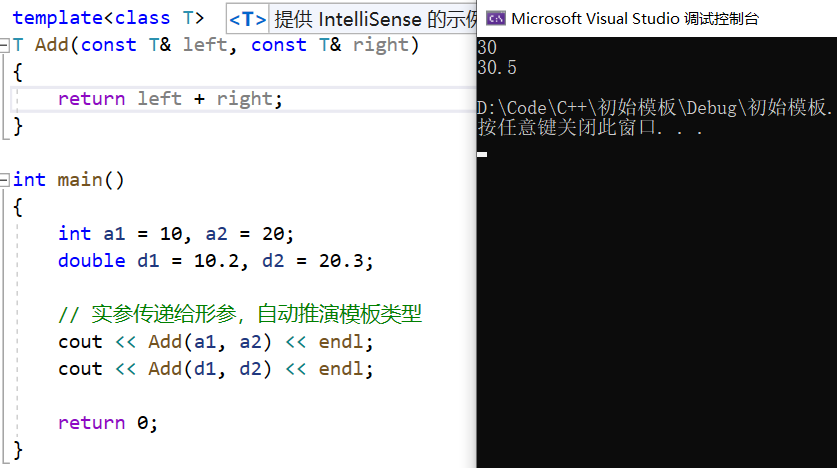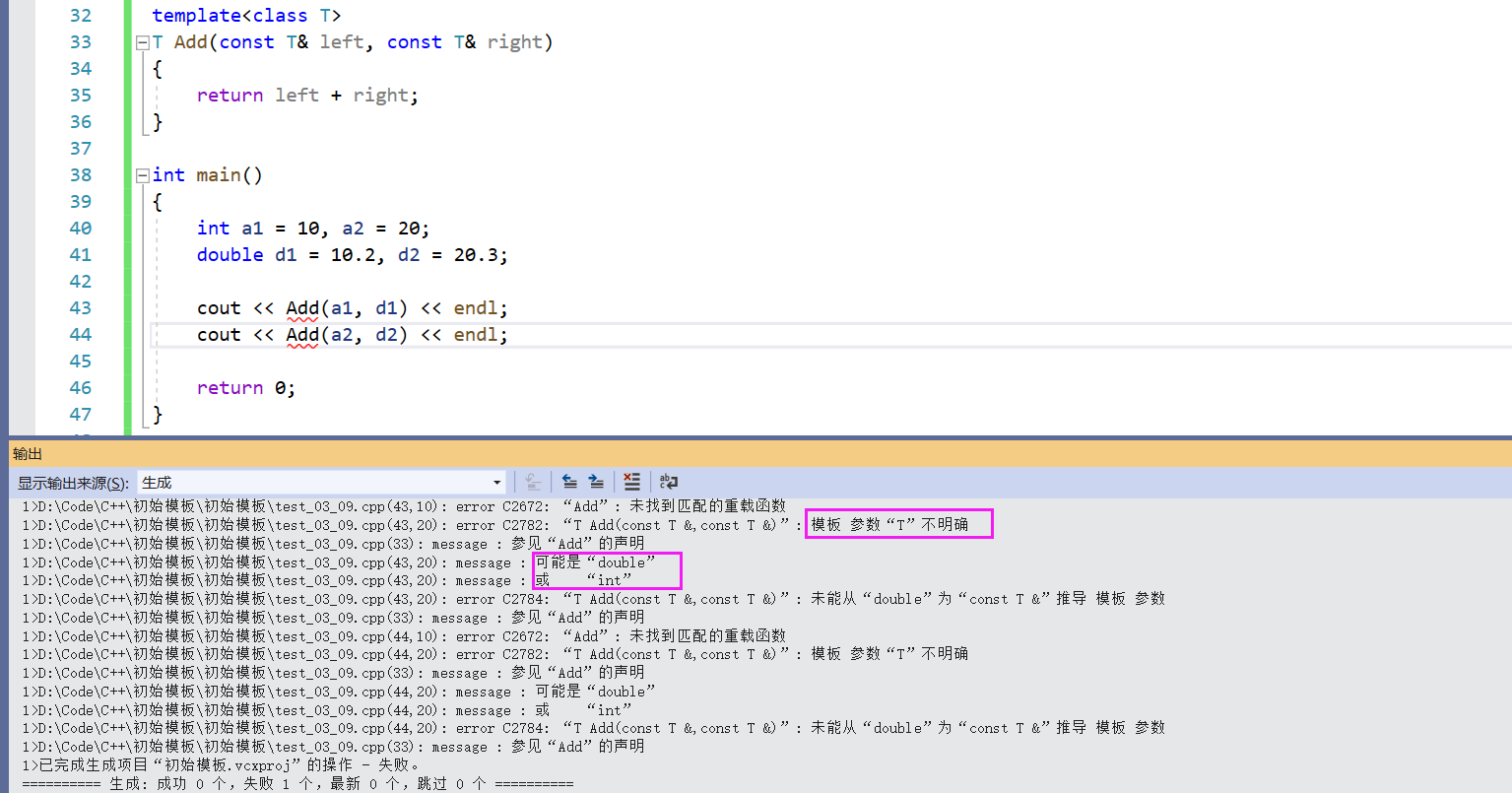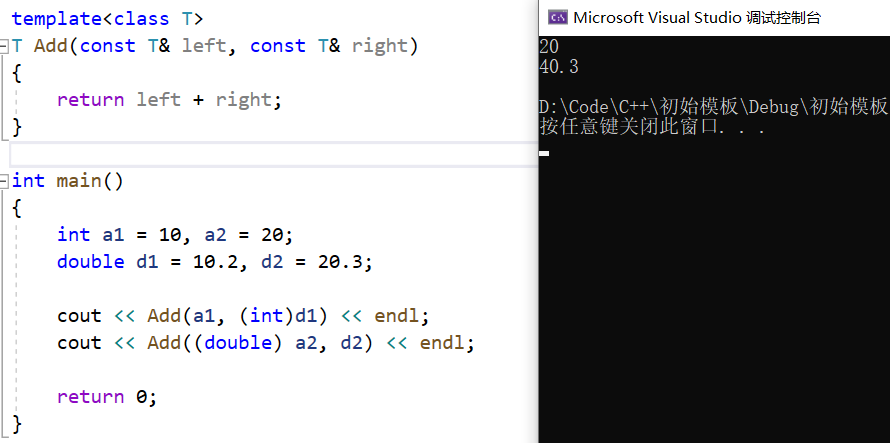• 显式实例化：在函数名后的<>中指定模板参数的实际类型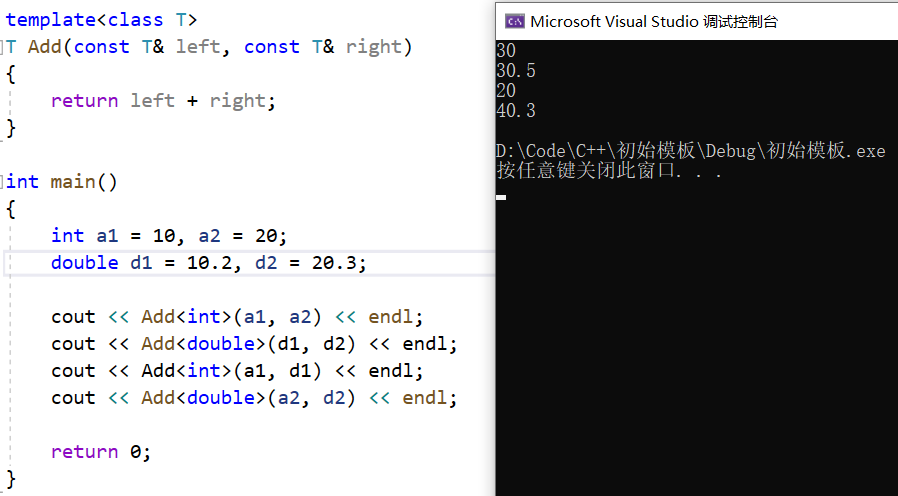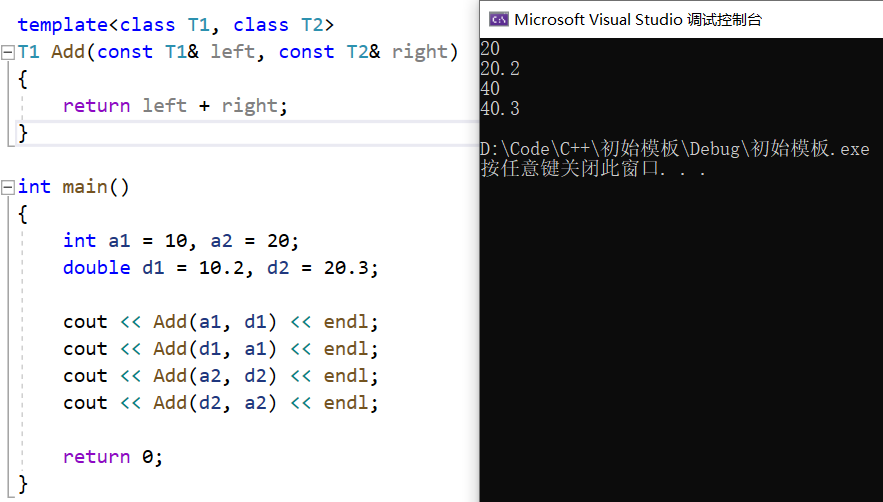#### 2.3 模板参数的匹配原则

• 一个非模板函数可以和一个同名的函数模板同时存在，而且该函数模板还可以被实例化为这个非模板函数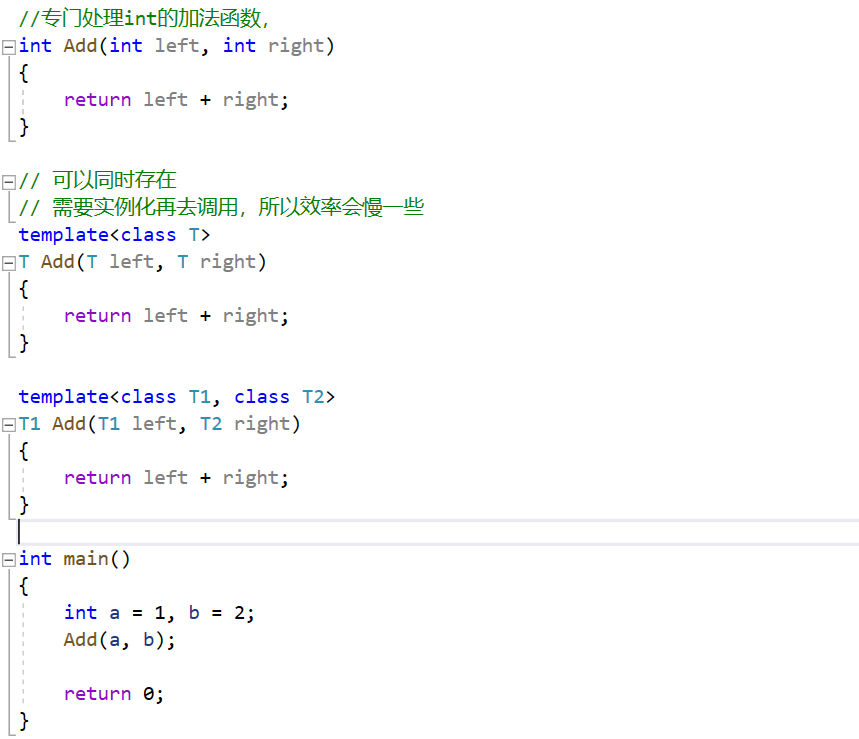• 对于非模板函数和同名函数模板，如果其他条件都相同，在调动时会优先调用非模板函数而不会从该模板产生出一个实例。如果模板可以产生一个具有更好匹配的函数， 那么将选择模板。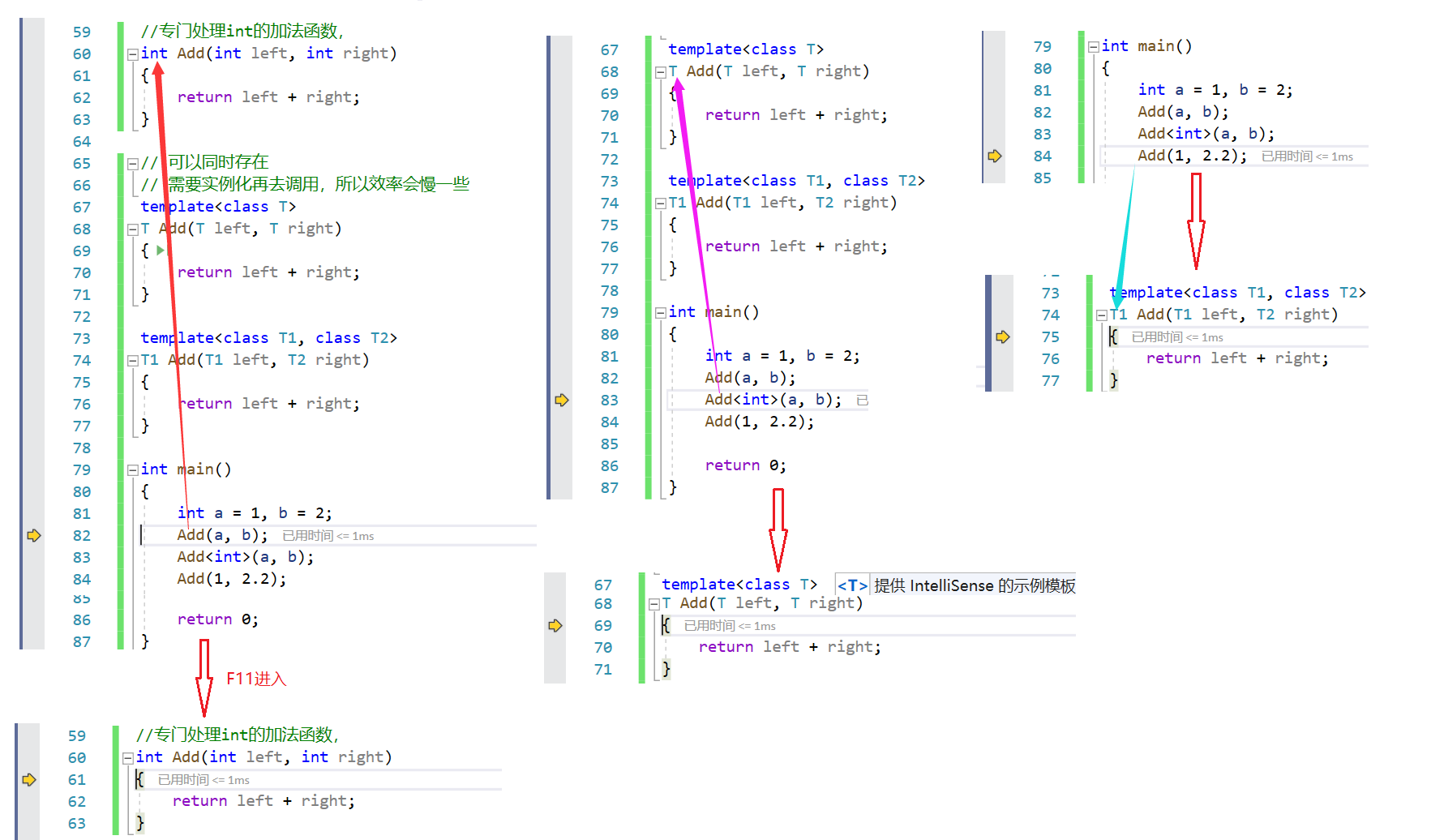• 模板函数不允许自动类型转换，但普通函数可以进行自动类型转换

### 三、类模板

`template<class T1, class T2, ..., class Tn> class 类模板名 { // 类内成员定义 };`

``````typedef double STDataType;

class Stack
{
public:
Stack(size_t capacity = 0)
{
_a = new T;
_top = 0;
_capacity = capacity;
}

~Stack()
{
delete[] _a;
_capacity = _top = 0;
}

private:
STDataType* _a;
size_t _top;
size_t _capacity;
};
``````

#### 3.1 类模板的实例化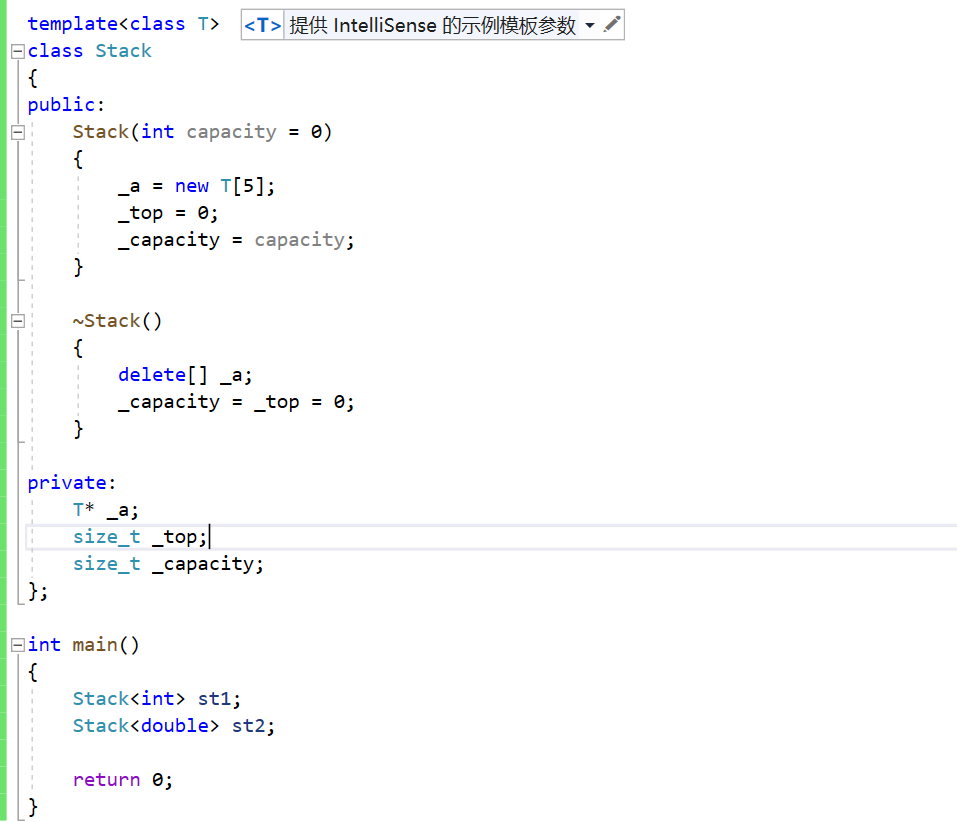#### 3.2 类模板与模板类``````// 注意：Vector不是具体的类，是编译器根据被实例化的类型生成具体类的模具
template<class T>
class Vector
{
public :
Vector(size_t capacity = 10)
: _pData(new T[capacity])
, _size(0)
, _capacity(capacity)
{}
// 使用析构函数演示：在类中声明，在类外定义。
~Vector();
void PushBack(const T& data)；
void PopBack()；
// ...
size_t Size() {return _size;}
T& operator[](size_t pos)
{
assert(pos < _size);
return _pData[pos];
}
private:
T* _pData;
size_t _size;
size_t _capacity;
};

// 注意：类模板中函数放在类外进行定义时，需要加模板参数列表
template <class T>
Vector<T>::~Vector()
{
if(_pData)
delete[] _pData;
_size = _capacity = 0;
}

int main()
{
Vector<int> v1; // Vector类名，Vector<int>才是类型
Vector<double> v2;

return 0;
}
``````

1.如果一个类被声明为类模板，那么其中的所有成员函数都是模板函数。
2.类模板中函数放在类外进行定义时，需要加模板参数列表。
3.模板的定义与声明不能分离！！！(这点我们以后再来探讨)。

### 【Java】基础练习（十）

1.判断邮箱 输入一个电子邮箱&#xff0c;判断是否是正确电子邮箱地址。 正确的邮箱地址&#xff1a; 必须包含 字符&#xff0c;不能是开头或结尾必须以 .com结尾和.com之间必须有其他字符 (1) Email类&#xff1a; package swp.kaifamiao.codes.Java.d0823; /** 输入一个…

### 基于广义神经网络的网络入侵检测Matlab代码

1.案例背景 1.1 FCM 聚类算法 聚类方法是数据挖掘中经常使用的方法,它将物理的或抽象的对象分为几个种群,每个种群内部个体间具有较高的相似性,不同群体内部间个体相似性较低。模糊c均值聚类算法(Fuzzy C- Mean, FCM)是用隶属度确定每个元素属于某个类别程度的一种聚类算法&am…

### 界面组件DevExpress Reporting——增强的SQL和实体框架数据源引入

DevExpress Reporting是.NET Framework下功能完善的报表平台&#xff0c;它附带了易于使用的Visual Studio报表设计器和丰富的报表控件集&#xff0c;包括数据透视表、图表&#xff0c;因此您可以构建无与伦比、信息清晰的报表。 本文总结了v23.1中针对DevExpress报表和BI Das…

### UI位置与布局

UI位置与布局 引言 发现UGUI的RectTransform定位还是很复杂的&#xff0c;感觉有必要详细了解一下 RectTransform 继承自Transform。他的local position由其他几个变量控制。建议不要直接设置position 目的是为了实现UI自动布局。这套方法将绝对定位&#xff0c;相对定位&a…

### React基础入门之虚拟Dom

React官方文档&#xff1a;https://react.docschina.org/ 说明 重要提示&#xff1a;本系列文章基础篇总结自尚硅谷课程&#xff0c;且采用类式写法&#xff01;&#xff01;最新的函数式组件写法见高级篇。 本系列文档旨在帮助vue同学更快速的学习react&#xff0c;如果你很…